Checkout JEE MAINS 2022 Question Paper Analysis : Checkout JEE MAINS 2022 Question Paper Analysis :

# Important Questions for Class 11 Chemistry Chapter 6 – Chemical Thermodynamics.

Class 11 chemistry important questions with answers are provided here for Chapter 6 – Chemical Thermodynamics. These important questions are based on the CBSE board curriculum and correspond to the most recent Class 11 chemistry syllabus. By practising these Class 11 important questions, students will be able to quickly review all of the ideas covered in the chapter and prepare for the Class 11 Annual examinations as well as other entrance exams such as NEET and JEE.

Download Class 11 Chemistry Chapter 6 – Chemical Thermodynamics Important Questions with Answers PDF by clicking on the button below.

## Class 11 Chemical Thermodynamics Important Questions with Answers

1. 18.0 g of water completely vapourises at 100°C and 1 bar pressure and the enthalpy change in the process is 40.79 kJ mol–1. What will be the enthalpy change for vapourising two moles of water under the same conditions? What is the standard enthalpy of vapourisation for water?

Given that, the amount of water is 18.0 g, and the pressure is 1 bar.

As we all know, 18.0 g H2O = 1 mole H2O.

Enthalpy change for vaporising 1 mole of H2O = 40.79 kJ mol–1

∴ Enthalpy change for vaporising 2 moles of H2O = 2 × 40.79 kJ = 81.358kJ

Standard enthalpy of vaporisation at 100℃ and 1 bar pressure,

Δvap H2O = + 40.79 k J mol–1.

2. One mole of acetone requires less heat to vapourise than 1 mol of water. Which of the two liquids has higher enthalpy of vapourisatIon?

Acetone requires less heat to vaporise due to the weak force of attraction between molecules.

As a result, water has a higher enthalpy of vaporisation.

It can be represented as:

Δvap(water) > Δvap (acetone)

3. Standard molar enthalpy of formation, ΔfHӨ is just a special case of enthalpy of reaction, ΔrHӨ. Is the ΔrHӨ for the following reaction same as ΔfHΘ ? Give reason for your answer. CaO(s) + CO2(g) → CaCO3(s); ΔfHӨ = –178.3 kJ mol–1

No, the ΔrHӨ for the given reaction is not same as ΔfHӨ.

The standard enthalpy change for the formation of one mole of a compound from its elements in their most stable states (reference states) is called standard molar enthalpy of formation, ΔrHӨ.

CaO(s) + C(s) + 3/2 O2(g) → CaCO3(s)

This reaction is different from the given reaction. Hence, ΔrHӨ ≠ ΔfHӨ.

4. The value of ΔfHӨ for NH3 is – 91.8 KJ mol–1. Calculate enthalpy change for the following reactIon:

2NH3 (g) → N2 (g) +3H2 (g)

ΔfHӨ is given for formation. For the reverse reaction, ΔfHӨ changes sign because the opposite of an exothermic reaction is an endothermic reaction.

So, for one mole, ΔfHӨ for decomposition is – (-91.8) = 91.8.

However, two moles are decomposing here.

Therefore, ΔfHӨ = 2 × 91.8 = 183.6 KJ mol–1.

5. Enthalpy is an extensive property. In general, if enthalpy of an overall reaction A→B along one route is ΔrH and ΔrH1, ΔrH2, Δr H3 ….. represent enthalpies of intermediate reactions leading to product B. What will be the relation between ΔrH for overall reaction and Δr H1 , Δr H2 ….. etc. for intermediate reactions.

In general, if enthalpy of an overall reaction A→B along one route is ΔrH and ΔrH1, ΔrH2, Δr H3 ….. represent enthalpies of reaction leading to same product B through another route, then we have ΔrH =ΔrH1 + ΔrH2 + Δr H3 +…..

For a general reaction Hess’s law of constant heat summation can be represented as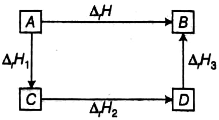6. The enthalpy of atomisation for the reaction CH4(g)→ C(g) + 4H (g) is 1665 kJ mol–1. What is the bond energy of C–H bond?

Enthalpy of atomisation of 4 moles of C−H bonds = 1665 kJ mol–1

∴ C−H bond energy, per mole = 1665 kJ mol–1/4 = 416.2 kJmol–1.

7. Use the following data to calculate Δlattice HӨ for NaBr.

Δsub HӨ for sodium metal = 108.4 kJ mol–1

Ionization enthalpy of sodium = 496 kJ mol–1

Electron gain enthalpy of bromine = – 325 kJ mol–1

Bond dissociation enthalpy of bromine = 192 kJ mol–1

ΔfHӨ for NaBr (s) = – 360.1 kJ mol–1

According to Hess’s Law,

ΔfHӨ = Δsub HӨ + ΔIEHӨ + ΔdissHӨ + ΔtgHӨ + U

Δsub HӨ for Na metal = 108.4 kJ/mol

I.E. of Na = 496 k/mol

ΔtgHӨ of Br = – 325 kJ mol–1

ΔdissHӨ of Br = 192 kJ mol–1

ΔfHӨ for NaBr = – 360.1 kJ mol–1

ΔfHӨ = Δsub HӨ + I.E. of Na + ΔdissHӨ + ΔtgHӨ + U

-360.1 = 108.4 +496 + 96 + (-325) – U

U = +735.5 kJ/mol

8. Given that ΔH = 0 for mixing of two gases. Explain whether the diffusion of these gases into each other in a closed container is a spontaneous process or not?

It is a spontaneous process. Although the change in enthalpy is zero, the change in randomness or disorder (i.e., ΔS) increases. As a result, in the equationΔG = ΔH – TΔS, the term TΔS will be negative. As a result, ΔG will be negative.

9. Heat has randomising influence on a system and temperature is the measure of average chaotic motion of particles in the system. Write the mathematical relation which relates these three parameters.

Heat has randomising influence on a system and temperature is the measure of average chaotic motion of particles in the system.

The mathematical relation which relates these three parameters is ΔS = qrev / T

Here, ΔS = change in entropy

qrev = heat of reversible reaction

T = temperature

10. Increase In enthalpy of the surroundings is equal to decrease In enthalpy of the system. Will the temperature of system and surroundings be the same when they are In thermal equilibrium?

Yes, when the system and the surroundings are in thermal equilibrium, their temperatures are same.

11. At 298 K. Kp for the reaction N2O4 (g) ⇄ 2NO2 (g) is 0.98. Predict whether the reaction is spontaneous or not.

For a spontaneous reaction, ΔrG° is -ve.

ΔrG° = – RT ln Kp = – RT ln (0.98)

In (0.98) is – 0.02

∴ΔrG° = – RT × –0.02

∴ Δ G°will be positive.

Hence, the reaction will be non spontaneous.

12. A sample of 1.0 mol of a monoatomic ideal gas is taken through a cyclic process of expansion and compression as shown in Fig. 6.1. What will be the value of ΔH for the cycle as a whole?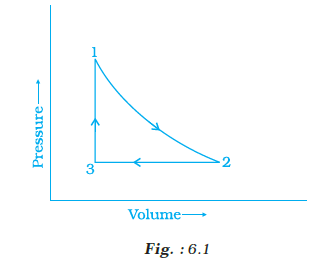The change in internal energy during a cyclic process is zero.

ΔU = 0

As the system returns to its initial state, no work is said to have been done.

Enthalpy for a steady-state cyclic process is one single value at any given stage, but it varies at different stages.

ΔH = 0

13. The standard molar entropy of H2O (l) is 70 J K–1 mol–1. Will the standard molar entropy of H2O (s) be more, or less than 70 J K–1 mol–1?

The solid form of H2O is ice. In ice, molecules are less random than in liquid water.

Thus, molar entropy of H2O (s) < molar entropy of H2O (l). The standard molar entropy of

H2O (s) is less than 70 J K–1 mol–1.

14. Identify the state functions and path functions out of the following: enthalpy, entropy, heat, temperature, work, free energy.

State Functions: Enthalpy, Entropy, Temperature, Free energy

Path Functions: Heat, Work

15. The molar enthalpy of vaporization of acetone is less than that of water. Why?

Acetone lacks hydrogen bond, so intermolecular forces are weaker, causing it to boil/evaporate quickly, lowering the molar enthalpy of vaporisation. Furthermore, because acetone lacks a polar O-H bond, it has a low enthalpy. Water has both a non-polar region and a strong hydrogen bond.

16. Which quantity out of ΔrG and ΔrGӨ will be zero at equilibrium?

ΔrG will always be zero.

ΔrGӨ is zero for K = 1 because ΔrGӨ = – RT ln K

ΔrGӨ will be non zero for other values of K.

17. Predict the change in internal energy for an isolated system at constant volume.

There is no energy transfer as heat or work in an isolated system,

so w=0 and q=0.

According to the first law of thermodynamics-

Δ U = q + w = 0 + 0 = 0

ΔU = 0

18. Although heat is a path function but heat absorbed by the system under certain specific conditions is independent of path. What are those conditions? Explain.

(i) At constant volume-

By first law of thermodynamics: q = ΔU + (–w)

(–w) = pΔV

∴ q = ΔU + pΔV

ΔV = 0, since volume is constant.

∴ qV = ΔU + 0 ⇒ qV = ΔU = change in internal energy

(ii) At constant pressure

qp = ΔU + pΔV

But, ΔU + pΔV = ΔH

∴ qp = ΔH = change in enthalpy.

So, at a constant volume and at constant pressure heat change is a state function because it is equal to change in internal energy and change in enthalpy respectively which are state functions.

19. Expansion of a gas in vacuum is called free expansion. Calculate the work done and the change in internal energy when 1 litre of ideal gas expands isothermally into vacuum until is total volume is 5 litre?

(–w) = pext (V2 – V1) = 0 × (5 –1) = 0

For isothermal expansion q = 0

By first law of thermodynamics-

q = ΔU + (–w)

0 = ΔU + 0

so, ΔU = 0

20. Heat capacity (Cp) is an extensive property but specific heat (c) is an intensive property. What will be the relation between Cp and c for 1 mol of water?

For water, heat capacity = 18 × specific heat

or Cp = 18 × c

Specific heat, c = 4.18 Jg–1 K–1

Heat capacity, Cp = 18 × 4.18 JK–1 = 75.3 J K–1.

21. The difference between Cp and Cv can be derived using the empirical relation H = U + pV. Calculate the difference between Cp and Cv for ten moles of an ideal gas.

For 1 mole of gas

Cp – Cv = R

For n moles of gas the relation is Cp – Cv = nR = 10 × 4.184 J

Cp – Cv = 41.84 J.

22. If the combustion of 1g of graphite produces 20.7 kJ of heat, what will be molar enthalpy change? Free the significance of sign also.

Molar enthalpy change of graphite = enthalpy change for 1g carbon ×

molar mass of carbon

ΔH = – 20.7 kJ g–1 × 12 g mol–1

ΔH = – 2.48 × 102 kJ mol–1.

The exothermic nature of the reaction is indicated by the negative sign of ΔH.

23. The net enthalpy change of a reaction is the amount of energy required to break all the bonds in reactant molecules minus amount of energy required to form all the bonds in the product molecules. What will be the enthalpy change for the following reaction.

H2(g) + Br2(g) → 2HBr(g)

Given that Bond energy of H2, Br2 and HBr is 435 kJ mol–1, 192 kJ mol–1 and 368 kJ mol–1 respectively.

ΔrHӨ = Bond energy of H2 + Bond energy of Br2 – 2 × Bond energy of HBr

ΔrHӨ= 435 + 192 – (2 × 368) kJ mol–1

ΔrHӨ = –109 kJ mol–1.

24. The enthalpy of vapourisation of CCl4 is 30.5 kJ mol–1. Calculate the heat required for the vapourisation of 284 g of CCl4 at constant pressure. (Molar mass of CCl4 = 154 g mol–1).

qp = ΔH = 30.5 kJ mol–1

∴ Heat required for vapourisation of 284 g of CCl4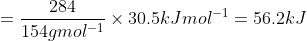25. The enthalpy of reaction for the reaction:

2H2 (g) + O2 (g) → 2H2O (l) is ΔrHΘ = – 572 kJ mol–1.

What will be standard enthalpy of formation of H2O (l )?

The enthalpy change for the following reaction will be the standard enthalpy of formation of H2O, according to the definition of standard enthalpy of formation.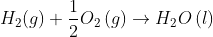or the standard enthalpy of formation of H2O (l) will be half of the given equation’s enthalpy, i.e., ΔrHΘ is also halved.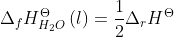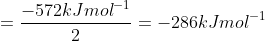26. What will be the work done on an ideal gas enclosed in a cylinder, when it is compressed by a constant external pressure, pext in a single step as shown in Fig. 6.2. Explain graphically.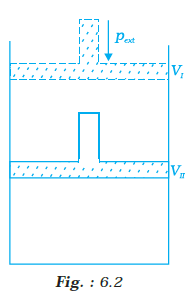Work done on an ideal gas can be calculated from p-V graph shown in Fig. 6.6. Work done is equal to the shaded area ABVIVII.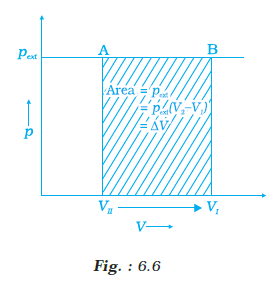.

27. How will you calculate work done on an ideal gas in a compression, when change In pressure Is carried out in infinite steps?

A process or change is said to be reversible if it is carried out in such a way that the process can be reversed at any time by an infinitesimal change.

When pressure is not constant and changes occur in infinite steps (reversible conditions) during compression from initial volume, Vi to final volume, Vf, work done can be calculated using a pV-plot. The shaded area represents the work done on the gas.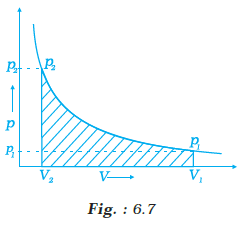28. Represent the potential energy/enthalpy change in the following processes graphically.

(a) Throwing a stone from the ground to roof.

(b) ½ H2(g) + ½ Cl2(g) ⇄ HCl(g); ΔrHΘ= –92.32 kJ mol–1

In which of the processes potential energy/enthalpy change is contributing factor to the spontaneity?

(a) When throwing a stone from the ground to the roof, we must provide energy to the stone.

(b) When heat is produced in a reaction, it indicates that the process is followed by a decrease in energy.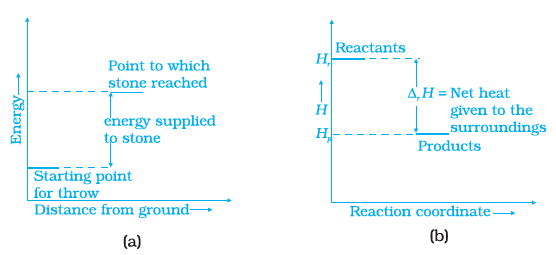Energy increases in (a) part while decreasing in (b). As a result, in process (b), the enthalpy change is a factor that contributes to the spontaneity.

29. Enthalpy diagram for a particular reaction is given in Fig. 6.3. Is it possible to decide spontaneity of a reaction from given diagram. Explain.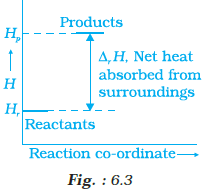No, enthalpy is not the only criterion for spontaneity; we must also consider entropy.

30. 1.0 mol of a monoatomic ideal gas is expanded from state (1) to state (2) as shown in Fig. 6.4. Calculate the work done for the expansion of gas from state (1) to state (2) at 298 K.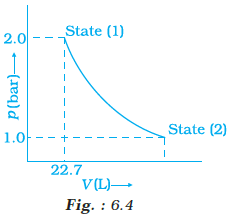Since the process is carried out in an infinite number of steps, it is clear from the diagram that it is a reversible change.

$$\begin{array}{l}w=-2.303RT log \frac{V_{2}}{V_{1}}\end{array}$$

$$\begin{array}{l}\frac{V_{2}}{V_{1}}=\frac{p_{1}}{p_{2}}=\frac{2}{1}=2\end{array}$$

$$\begin{array}{l}w=-2.303RT log\frac{p_{1}}{p_{2}}\end{array}$$

$$\begin{array}{l}w=-2.303\times 8.314Jmol^{-1}\times 298K^{-1}\times log2\end{array}$$

= -1717.46 J

31. An ideal gas is allowed to expand against a constant pressure of 2 bar from 10 L to 50 L in one step. Calculate the amount of work done by the gas. If the same expansion were carried out reversibly, will the work done be higher or lower than the earlier case? (Given that 1 L bar = 100 J).

Work done in the proess can be calculated as-

w = –Pex (Vf – Vi)

w = – 2 × 40 = – 80 bar = – 8 kJ

The negative sign indicates that the system is working on the environment. Because internal and external pressures are nearly equal at every step, the work done will be more in the reversible expansion.

1. Derive the relationship between ΔH and ΔU for an ideal gas. Explain each term Involved in the equation.

When heated, the volume of solids and liquids does not change significantly. As a result, if the volume changes, ∆V is insignificant.

∆H = ∆U + P∆V

∆H = ∆U + P(0)

∆H = ∆U

When gases are involved in the reaction, the difference between the change in internal energy and the change in enthalpy becomes significant.

Consider a chemical reaction that takes place at a constant temperature, T, and pressure, P. Let’s say the reactants’ volume is VA and the number of moles in the reactants is nA. Similarly, the product volume is VB, and the number of moles in the product is nB.

We know from the ideal gas equation that-

pV = nRT

pVA = nART

pVB = nBRT

Thus,

pVA – pVB = nART – nBRT

p(VA – VB)= RT(nA – nB)

p∆V = ∆ngRT

∆H = ∆U + p∆V

∆H = ∆U + ∆ngRT

2. Extensive properties depend on the quantity of matter but intensive properties do not. Explain whether the following properties are extensive or intensive.

Mass, internal energy, pressure, heat capacity, molar heat capacity, density, mole fraction, specific heat, temperature and molarity.

• Mass is an extensive property because it is dependent on the amount of matter.
• Internal energy is proportional to volume, making it an extensive property.
• Because pressure is independent of the amount of substance, it is an intensive property.
• Heat capacity is an extensive property, whereas molar heat capacity is an intensive property because it is the ratio of two extensive properties.
• Density is an intensive property because it is the ratio of two extensive properties.
• Since mole fraction is the ratio of two extensive properties, it is an intensive property.
• Specific heat is an intense property because it is independent of the amount of substance.
• An intensive property is a ratio of two extensive properties therefore molarity is an intensive property.
• Temperature is also an intensive property as it is independent of the amount of substance.

3. The lattice enthalpy of an ionic compound is the enthalpy when one mole of an ionic compound present in its gaseous state, dissociates into its ions. It is impossible to determine it directly by experiment. Suggest and explain an indirect method to measure lattice enthalpy of NaCl(s).

When one mole of an ionic compound dissociates into its ions, the enthalpy change related to this process is called lattice enthalpy.

Na+Cl (s) → Na+ (g) + Cl (g); ∆latticeHΘ = + 788 hJmol–1

Lattice enthalpy for NaCl can be calculated as follows-

i. Sublimation of sodium metal

Na (s) → Na (g), ∆subHΘ = 108.4 kJ mol–1

ii. Ionisation of sodium atoms

Na(g) → Na+ (g) + e (g); ∆tHΘ = 496 kJ mol–1

iii. Dissociation of chlorine molecule, the reaction is half the bond dissociation enthalpy.

½ Cl2 (g) → Cl (g); ½ ∆bondHΘ = 121 kJmol–1

iv. Cl (g) + e (g) → Cl (g); electron gained by chlorine atoms. The electron gain enthalpy, ∆egHΘ = – 348.6 kJ mol–1.4. ΔG is net energy available to do useful work and is thus a measure of “free energy”. Show mathematically that ΔG is a measure of free energy. Find the unit of ΔG. If a reaction has positive enthalpy change and positive entropy change, under what condition will the reaction be spontaneous?

∆STotal = ∆Ssys + ∆Ssurr

∆STotal = ∆Ssys + ( – ∆Hsys/T)

T∆STotal = T∆Ssys – ∆Hsys

For spontaneous change, ∆STotal > 0

∴ T∆Ssys – ∆Hsys > 0

-(∆Hsys – T∆Ssys) > 0

But, ∆Hsys – T∆Ssys = ∆Gsys

∴ –∆Gsys > 0

∆Gsys = ∆Hsys – T∆Ssys < 0

∆Hsys = Enthalpy change of a reaction

T∆Ssys = Energy which is not available to do useful work

∆Gsys = Energy available for doing useful work

• If ∆G is negative (< 0), the process is spontaneous.
• If ∆G is positive (> 0), the process is non spontaneous.

Unit of ∆G is Joule.

The reaction will be spontaneous at high temperature.

5. Graphically show the total work done in an expansion when the state of an ideal gas is changed reversibly and isothermally from (pi, Vi ) to (pf, Vf ). With the help of a pV plot compare the work done in the above case with that carried out against a constant external pressure pf.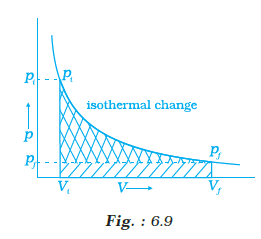(i) Reversible Work is represented by the combined areas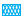and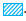(ii) Work against constant pressure, pf is represented by the areaWork (i) > Work (ii)